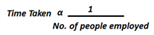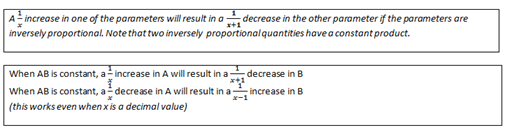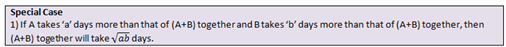# Time & Work: Efficiencies

This concept is a part of the time and work topic of the CAT quantitative aptitude section. Here the approaches to such questions are given along with a shortcut technique to help the CAT candidates solve the related questions efficiently.

### Time & Work- Equal Efficiencies:

#### Approach 1:

Using the concept of equal efficiencies:- When efficiencies are equal then the Time taken to do a certain work is always inversely proportional to the number of people employed.#### Approach 2:

We can use the concept of Man- Days. Every work to be done has a quantity attached to it. That quantity is the product of the number of men and the Time taken. So the quantity can be named as “MAN-HOURS” or “MAN – DAYS” or anything similar depending upon the question. For work, this quantity will always be constant irrespective of any change in the number of men or/and Time if we assume that the men are working with equal efficiency.

Example 1: If 25 men can finish the work in 12 days, 30 men can finish the same work in how many days?

#### Approach 1:

Men days 25 12 30 xi.e. (Time 1)/(Time 2) = (Men 2)/(Men 1) Less number of men means more number of days to complete the work, i.e. Men and days are inversely proportional. Therefore x/12=25/30 => x =10.

#### Approach 2:

Given that 25 men can finish the work in 12 days. So MAN-DAYS needed to complete the work = 25 * 12 = 300 MAN-DAYS. Even if we change the number of men working; for the completion of work we need 300 MAN-DAYS. When we have 30 men then the number of days taken = (MAN-DAYS)/(number of men) ➜ 300/30= 10 days.

#### Approach 3: Shortcut Technique

Using the Constant Product Rule: Since Man × days = man-days ( constant) 1/5 increase in the number of men (25 to 30) will result in 1/6 reduction in number of days => (1/6× 12 = 2 days). Answer= 12-2= 10 days.Example 2: Mahesh has an engineering firm. He receives a work order, which he estimates to finish in a certain Time by employing 50 men. But at the end of the 50th day, against his expectation that half of the work will be finished, only 40% of the work is finished. How many more men should he employ in order to complete the work in Time?

Solutions:

At the end of the 50th day he was expecting half of the work to be finished i.e., he aims to finish the work by the 100th day. 50 men worked for 50 days i.e. 2500 MAN-DAYS resulted in 40% completion of the work, so to complete the rest 60 % he needs 2500×0.6/0.4 = 3750 MAN-DAYS.

Now, to finish the work he has 50 days left and the requirement is 3750 MAN-DAYS, so he needs 3750/ 50 = 75 men to work i.e. he needs to employ 25 more men.

### Time & Work- Unequal Efficiencies:

• #### Questions Where Different People Work

These are cases including:

A works for the first 2 days, B works for the next 2 days and C works till the finish. Find the Time taken

Pipe A is open for 3 hours, Pipe B for 2 hours and Pipe C for 1.5 hours. Find the number of hours in which the cistern is filled.

### Illustrations:

1) A frog falls into a well and is trying to come out. Every day he is able to cover 10% of the total height of the well in the morning, but during the night, he slips down by 5% of the total height of the well. When will the frog be able to come out of the well?

Solution:

During the day Time he climbs up 10% & during the night he slips down by 5%. So in a full day, he climbs up by a net 5%.

Hence, in 18 full days, he will cover 90% of the well. He will complete the remaining 10% the next day Total= 19 days

You may tend to choose 20 days as your answer as the general tendency will be to calculate the net change in a day i.e. 5%. So to cover 100%, it should take 100/5= 20 days.

Try another question based on the same logic.

2) There are two inlet pipes A and B. A fills the tank in 8 hrs and B in 12 hrs. They have opened alternatively for an hour each starting with A at 10 am. When will the tank be filled?

Solution:

A takes 8 hrs so in one hour it will fill 100/8= 12.5% of the tank. B takes 12 hours so in one hour it will fill 100/12= 8.33% of the tank. So in two hours, they will fill = 20.83% of the tank. At the end of 8 hours they fill 20.83 * 4= 83.33% of the tank Percentage of tank unfilled = 100-83.33= 16.67 At the end of 9 hours, tank unfilled = 16.67-12.5 ≈ 4% So to complete the last 4 %, it will take 8.33/4≈ 0.5 hours = 30 minutes.

So tank will be filled by around 7:30 pm3) A takes 9 days more than that of A and B together to finish a work, B takes 4 days more than that of A and B together to finish the work, then A and B together will take how many days to finish the work?

Solution:

Using the formula given above we can directly find out the answer as √9×4= √36 =6 days.

4) Among four persons A, B, C and D, C works half as fast as A while D works a third as fast as B. If C and D, when working individually, complete the work in 24 and 54 days respectively more than the Time in which they complete the work when working together, then find the Time in which A and B, working together, will complete the work?

Solution:

C and D complete the work in 24 and 54 days more than the number of days they take to complete the work together

Thus, Together they will complete the work in (24 x 54)1/2 = 36 i.e.C will take 36 + 24 = 60 days; In one day: 100/60 of the work is done

Since A is two times faster than C, he will take 30 days

D takes 54 days more i.e. he will take 36 + 54 = 90 days. In one day: 100/90 of the work is done Since B works 3 Times faster than D, he will take 30 days

A + B together =100/30+100/30=100/15 i.e. Together they will finish the job in 15 days.

• #### Questions where People leaves or joins a work

These are cases including:

✔ A and B working on a project. B leaves after n days, how long does A take to complete the project?

✔ A and B working on a project. B leaves after n days, C joins in, so how long do A & C take to complete the project together?

✔ There are n taps in a tank. Initially, tap 1 is open, after an hour, tap 2 is open and so on. Find the Time taken to fill the tank

The fastest way to solve these types of questions is to use the “percentage” concept. Let us look at a few examples solved in this way.

### Illustrations:

1) A can do a work in 18 days, B in 9 days and C in 6 days. A and B start working together and after 2 days C joins them. In how many days will the job be finished?

solution:-

In one day A can do 100/18 % of the work= 5.55%

In one day B can do 100/9 % of the work= 11%

In one day C can do 100/6 % of the work= 16.67%

A and B start together and work for two days.

Work done by A and B in one day = 100/18+100/9= 16.67%

Work done by A and B in 2 days = 2 * 16.67 % = 33.34 %

So when C joins them work left to be done = 66.67%

In one day A+ B + C can do 33.33% of work.

So to complete the left 66.67 %, the three of them need to work for two more days. So the job will be finished in 4 days.

2) Ramesh can do a piece of work in 10 days, Mahesh in 12 days and Arpita in 15 days. They all start together but Ramesh leaves after 2 days and Mahesh leaves 3 days before the work is completed. In how many days is the work completed.

Solution:

Ramesh can do 100/10%= 10% of work in a day.

Mahesh can do 100/12% = 8.33% of work in a day.

Arpita can do 100/15% = 6.66% of work in a day.

They both start together and Ramesh leaves after 2 days. So in the two days that they work together they will complete 2×(10+8.33+6.66) % of work = 2 × 25 % = 50 %. After Ramesh leaves, 50 % of the work is left. That is done by Mahesh and Arpita.

But 3 days before the completion of work Mahesh leaves ➜Arpita completes the work in three days alone. In last three days work done by Arpita = 3 × 6.66% = 20 %.This means 30 % of the work is done by Mahesh and Arpita together. They together can do (8.33+ 6.66) = 15 % of work in a day. So to complete 30 % of the work they would have worked 2 days. So the work is completed in 2 + 2 + 3 = 7 days.

### Time & Work- Operational Efficiencies.

• #### Efficiencies are equal within a group

This can be understood better with the following example.

Question: 2 men and 7 boys can do a piece of work in 14 days. 3 men and 8 boys can do the same piece of work in 11 days. Then 8 men and 6 boys can do 3 Times the work in how many days?

Solution:

Two steps are to be followed:

i) Find out the relation between the work done by a man and that by a boy. For this equate the work done in 1 day by both groups

ii) Once you have the relation from step (i) convert the group into groups of only men or only boys. This way you will be in a position to compare two groups easily.

2 men and 7 boys are needed to do a piece of work in 14 days. This means to complete the work we need 2 men to work for 14 days and 7 boys to work for 14 days. So if we want to complete the work in one day we need: 2(14) = 28 men and 7(14) = 98 boys => 28M + 98B.

Also, 3 men and 8 boys take 11 days, so if they need to complete the work in 1 day, we need 3(11) =33 men and 8 (11) =88 boys => 33M + 88B

The two cases can now be equated as 28M + 98B = 33M + 88B

Solving them, one gets 5M=10B or 1M=2B i.e. one man is equivalent to two boys.

Converting both the groups as a group of only men:

3 men & 8boys = 3men + 4 men = 7 men can finish one work in 11 days

The question is for a group of 8 men and 6 boys which equate to 8 + 6/2 Men = 11 Men.

If 7 men take 11 days, then 11 men will take 7 days to finish one of the work.

To finish 3 Times the work, they will take 7×3=21 days.Visit time and work questions to practice more similar questions. Stay tuned with BYJU’S to get more such shortcut techniques and tips for different CAT exam topics.